# ML Aggarwal Class 7 Solutions for ICSE Maths Chapter 15 Visualising Solid Shapes Objective Type Questions

## ML Aggarwal Class 7 Solutions for ICSE Maths Chapter 15 Visualising Solid Shapes Objective Type Questions

Mental Maths

Question 1.
Fill in the blanks:
(i) A solid having no vertex and no edge is a ………..
(ii) A solid that has congruent and parallel polygons as top and bottom faces and all other faces rectangular is known as ………
(iii) A pyramid having 4 equilateral triangles as its faces is known as ………
(iv) A solid having 3 faces (one curved and two circulars), no vertex and two curved edges are known as ……..
(v) A solid having a circular base and one vertex is called a ………
(vi) A triangular prism has ……… faces, ………… edges and ………. vertices.
(vii) A triangular pyramid has ……… faces, ………. edges and ……… vertices.
(viii) A square pyramid has ……… faces, ……….. edges and ……… vertices.
(ix) The base of a triangular pyramid is a ………
(x) Out of ……….. faces of a triangular prism, ……… are rectangle and ……….. are triangles.
(xi) Out of ……….. faces of a square pyramid, ………. are a triangle and ………. is/are squares.
(xii) Out of ………. faces of a rectangular pyramid, ………. are triangles and the base is a ……….
(xiii) A ……….. is a sort of skeleton – outline in 2-D, which on folding results in a 3-D shape.
(xiv) If the sum of numbers on the two dice thrown together is 9, then the sum of the numbers opposite to these faces is ……….
Solution: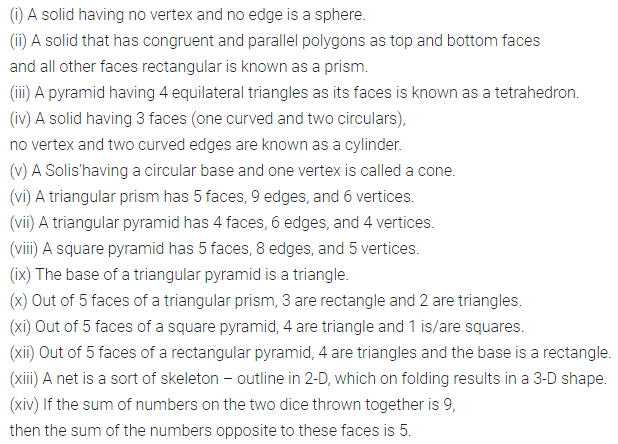Question 2.
State whether the following statements are true (T) or false (F):
(i) The faces of a prism are triangular.
(ii) A cube can be treated as a prism.
(iii) A pyramid has only one vertex.
(iv) All the faces, except the base, of a square pyramid are triangular.
(v) A tetrahedron has 3 rectangular faces and 1 rectangle face.
(vi) A square pyramid has 5 faces and one vertex.
(vii) A cone has one vertex, two faces, and one curved edge.
(viii)The shadow of a 3-D object is a 2-D figure.
(ix) A cube can cast a shadow in the shape of a rectangle.
(x) A cube can cast a shadow in the shape of a hexagon.
(xi) In an isometric sketch, the line segments of different lengths can represent the sides of a cube.
(xii) In an oblique sketch of a cuboid, the size of the opposite faces must be different.
(xiii) The top, front, and side views of a sphere are different.
(xiv) The adjoining net is of a hexagonal pyramid.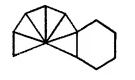Solution: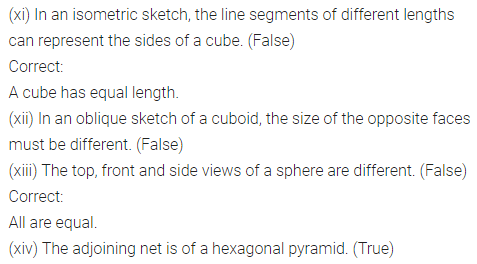Multiple Choice Questions

Choose the correct answer from the given four options (3 to 11):
Question 3.
A triangular prism has
(a) 4 vertices and 6 edges
(b) 6 vertices and 9 edges
(c) 6 vertices and 6 edges
(d) 9 vertices and 6 edges
Solution: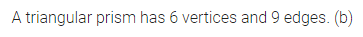Question 4.
A square pyramid has
(a) 4 vertices and 4 faces
(b) 4 vertices and 5 faces
(c) 5 vertices and 4 faces
(d) 5 vertices and 5 faces
Solution: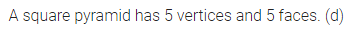Question 5.
A solid having 4 (plane) faces, 4 vertices and 6 edges is called a
(a) triangular prims
(b) rectangular prism
(c) triangular pyramid
(d) rectangular pyramid
Solution:Question 6.
The number of cubes in the given structure is
(a) 12
(b) 10
(c) 9
(d) 8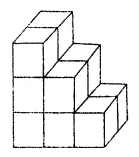Solution:Question 7.
An isometric sheet is made up of dots forming
(a) squares
(b) rectangles
(c) right-angled triangles
(d) equilateral triangles
Solution: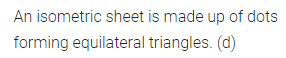Question numbers 8 to 11 are based on the given figure in which unit cubes are put together to form a structure as shown: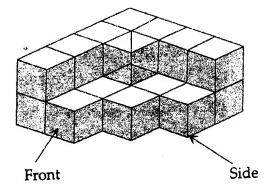Question 8.
The number of unit cubes in the given structure is
(a) 13
(b) 20
(c) 21
(d) 22
Solution:Question 9.
The number of unit cubes to be added to make a cuboid of dimensions 4 unit × 4 unit × 2 unit is
(a) 11
(b) 12
(c) 13
(d) 14
Solution:Question 10.
If the structure is painted on the surface everywhere, then the number of unit cubes having no face painted is
(a) 0
(b) 1
(c) 2
(d) 11
Solution: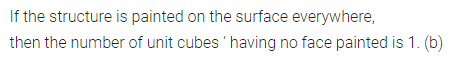Question 11.
The side view of the given structure isSolution: